#Python编程常用技巧，你知道几个？

0
0
01. 云栖社区>
2. 云栖号资讯>
3. 博客>
4. 正文

## Python编程常用技巧，你知道几个？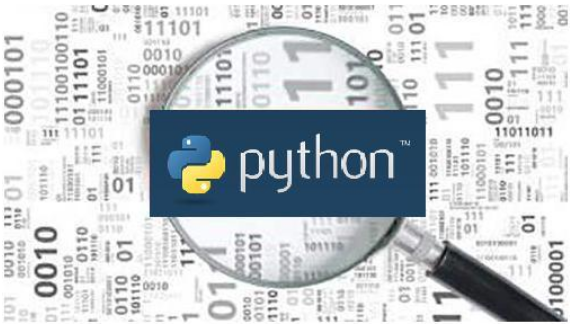1. 清理用户输入

user_input = "This\nstring has\tsome whitespaces...\r\n"

character_map = {
ord('\n') : ' ',
ord('\t') : ' ',
ord('\r') : None
}


2. 提示用户输入

import getpass

user = getpass.getuser()


3. 查找字符串频率

import difflib
difflib.get_close_matches('appel', ['ape', 'apple', 'peach', 'puppy'], n=2)
# 返回['apple', 'ape']


difflib.get_close_matches会查找相似度最匹配的字串。本例中，第一个参数与第二个参数匹配。提供可选参数n，该参数指定要返回的最大匹配数，以及参数cutoff(默认值为0.6)设置为thr确定匹配字符串的分数。

4. 多行字符串

Python中可以使用反斜杠：

In : multistr = " select * from test \
...: where id < 5"
In : multistr
Out: ' select * from test where id < 5'


In : multistr ="""select * from test
...: where id < 5"""
In : multistr
Out: 'select * test where id < 5'


In : multistr = ("select * from multi_row "
...: "where row_id < 5 "
...: "order by age")
In : multistr
Out: 'select * from multi_row where row_id < 5 order by age'


5. 处理IP地址

import ipaddress


＃192.168.1.0
＃192.168.1.1
＃192.168.1.2
＃192.168.1.3
＃...


ip = ipaddress.ip_address("192.168.1.2")
ip in net
# True

ip in net
# False

ip地址转字符串、整数值的互转：

>>> str(ipaddress.IPv4Address('192.168.0.1'))
'192.168.0.1'
3232235521
'::1'
1

1. 限制CPU和内存使用量

# CPU限制
def time_exceeded(signo, frame):
print("CPU 超额...")
raise SystemExit(1)
def set_max_runtime(seconds):
soft, hard = resource.getrlimit(resource.RLIMIT_CPU)
resource.setrlimit(resource.RLIMIT_CPU, (seconds, hard))
signal.signal(signal.SIGXCPU, time_exceeded)
# 限制内存使用
def set_max_memory(size):
soft, hard = resource.getrlimit(resource.RLIMIT_AS)
resource.setrlimit(resource.RLIMIT_AS, (size, hard))

2. 通过__slots__节省内存

import sys
class FileSystem(object):
def __init__(self, files, folders, devices):
self.files = files
self.folders = folders
self.devices = devices
print(sys.getsizeof( FileSystem ))

class FileSystem1(object):

__slots__ = ['files', 'folders', 'devices']
def __init__(self, files, folders, devices):
self.files = files
self.folders = folders
self.devices = devices
print(sys.getsizeof( FileSystem1 ))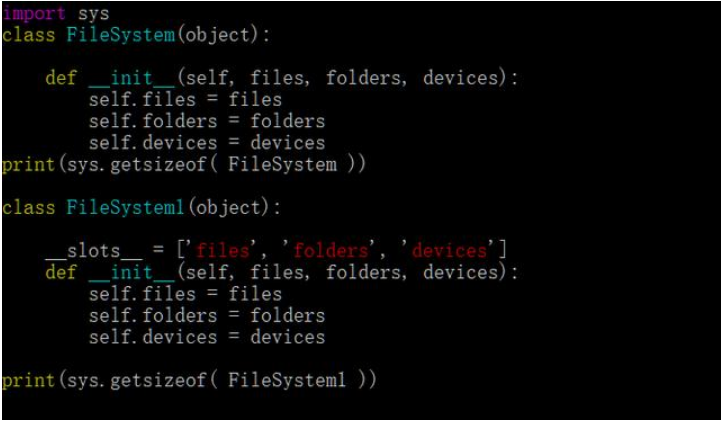# Python 3.5下
#1-> 1016
#2-> 888


3. 用lru_cache缓存函数调用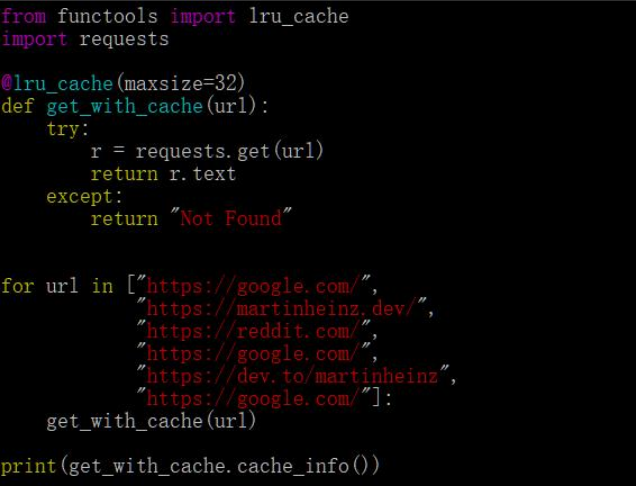# CacheInfo(hits=2, misses=4, maxsize=32, currsize=4)


4. __all__控制import

def foo():
pass
def bar():
pass
__all__ = ["bar"]


1. 创建支持With语句的对象

class Connection:
def __init__(self):
...
def __enter__(self):
# Initialize connection...
def __exit__(self, type, value, traceback):
# Close connection...
with Connection() as c:
# __enter__() executes
...
# conn.__exit__() executes


from contextlib import contextmanager

@contextmanager
def tag(name):
print(f"<{name}>")
yield
print(f"</{name}>")

with tag("h1"):
print("This is Title.")


2. 重载运算符号的技巧

from functools import total_ordering
@total_ordering
class Number:
def __init__(self, value):
self.value = value
def __lt__(self, other):
return self.value < other.value
def __eq__(self, other):
return self.value == other.value
print(Number(20) > Number(3))
print(Number(1) < Number(5))
print(Number(15) >= Number(15))
print(Number(10) <= Number(2))


3. 在一个类中定义多个构造函数

import datetime
class Date:
def __init__(self, year, month, day):
self.year = year
self.month = month
self.day = day
@classmethod
def today(cls):
t = datetime.datetime.now()
return cls(t.year, t.month, t.day)
d = Date.today()
print(f"{d.day}/{d.month}/{d.year}")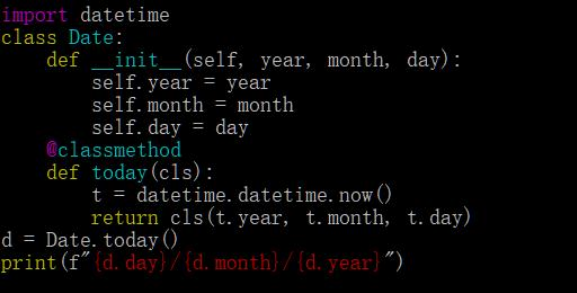# 14/9/2019


4. 获取对象信息

Python提供了几个函数以便我们更好的获取对象的信息，这些函数包括：type、isinstance和dir。

>>> type(None)
<class 'NoneType'>
>>> type(abs)
<class 'builtin_function_or_method'>


>>> type(11) == type(22)
True
>>> type('abc') == str
True
>>> type('abc') == type(33)
False


isinstance():可以显示对象是否是某种类型

>>> class Husty(Dog):
... pass
...
>>> a = Animal()
>>> b = Dog()
>>> c = Husty()
>>> isinstance(c,Husty)
True
>>> isinstance(c,Dog)
True
>>> isinstance(c,Animal)
True
>>> isinstance(b,Husty)
False


Husty是Husty、Dog、Animal类型的对象，却不能说Dog是Husty的对象。

dir():用于获取一个对象的所有方法和属性。返回值是一个包含字符串的list：

>>> dir('abc')
……'isalnum
'isidentifier', 'islower', …… 'translate', 'upper', 'zfill']


5. Iterator和切片

import itertools
s = itertools.islice(range(50), 10, 20)
for val in s:
...


6. 跳过一些行

string_from_file = """
// Author: ...
//
// Date: ...
Actual content...
"""

import itertools
for line in itertools.dropwhile(lambda line: line.startswith("//"), string_from_file.split("\n")):
print(line)


7. 命名切片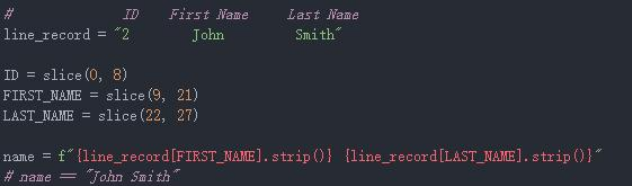1. 脚本调试

Python的脚本调试可以是使用pdb模块。它可以让我们在脚本中随意设置设置断点：

import pdb
pdb.set_trace()


2. 在shell中调试程序

def func():
return 0 / 0
func()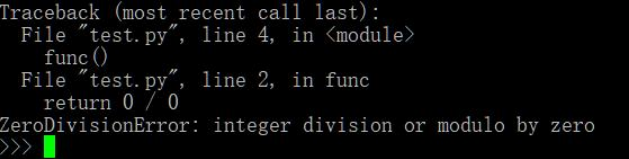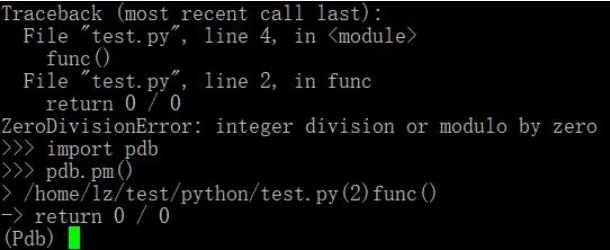import pdb;
def func():
pdb.set_trace()
return 0 / 0
func()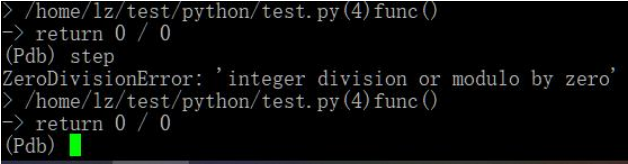1. 一键web服务共享

python -m http.server


python -m http.server 8888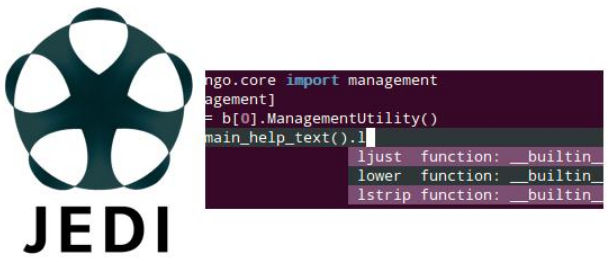Jedi是一个用于Python代码自动补齐和静态分析的库。Jedi可以让我们高效的敲代码。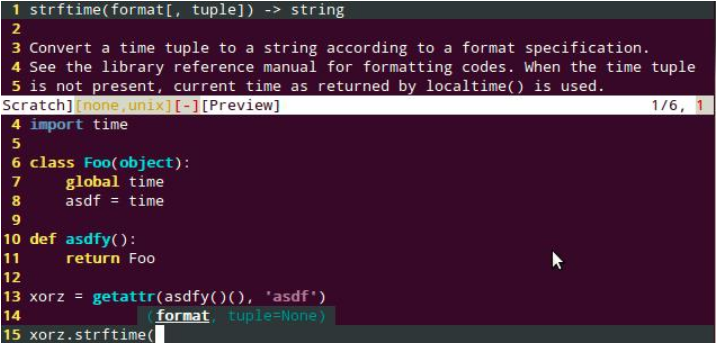2. 美化异常输出pretty-errors

Python默认的报错输出非常乱，看的人头大，可读性差。这时候就需要用pretty-errors这个错误美化工具了。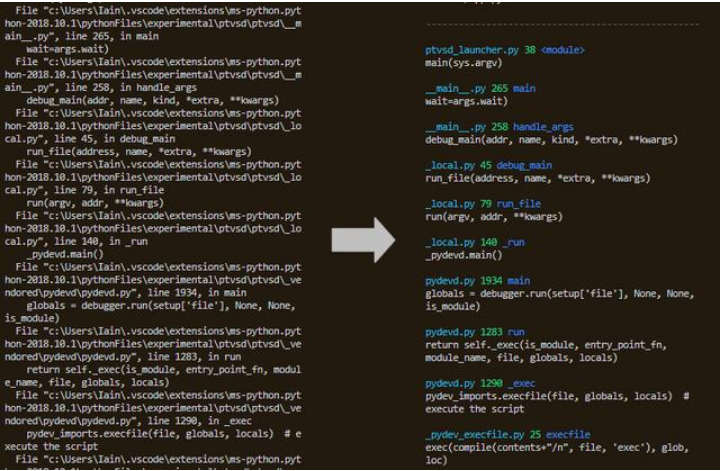+ 关注